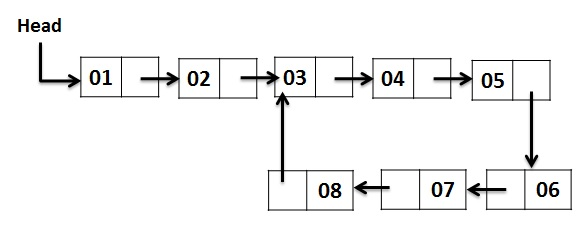Q:

# Find length of loop in a linked list

Given a linked list, write a program that checks whether the given Linked List contains a loop or not and if the loop is present then return the count of nodes in the loop or else return 0. (this is mainly a functional problem).

Example:There is a loop in the above linked list.
As, 1 → 2 → 3 → 4 → 5 → 6 → 7 → 8 → 3 → 4 → 5 → 6 → 7 → 8 → 3...so on.

Thus, there is a loop between 3 and 8 and the length of loop is 6.

The above problem has two sections:

1. Detecting loop in the linked list
2. Finding length of loop if loop exists

We declare two pointers: one slow pointer and another fast pointer. The slow pointer moves slowly, only one step at each iteration. The fast pointer moves fast, two steps at each iteration. Slow pointer and fast pointer are initially set to the head & head->next respectively.

If there is a loop then the fast pointer and slow pointer will point to a similar node after finite no of iteration
Else
The fast pointer will reach NULL.

Let’s solve the above example using this algorithm...

```    Initially both points to head node...

At iteration 0:
slow points to 2 (head->next )

At iteration 1:
slow points to 3 (only one traverse one node)
fast points to 5(traverse two nodes)

At iteration 2:
slow points to 4 (only one traverse one node)
fast points to 7  (traverse two nodes)

At iteration 3:
slow points to 5 (only one traverse one node)
fast points to 3 (traverse two nodes)

At iteration 4:
slow points to 6 (only one traverse one node)
fast points to 5  (traverse two nodes)

At iteration 5:
slow points to 7 (only one traverse one node)
fast points to 7 (traverse two nodes)

both points to same node, loop detected
```

In case there’s no loop, let’s consider these case too...

Such an example is any single linked list terminated by NULL, 1 → 2 → 3 → 4 → 5 → 6 → NULL

In this case the fast pointer reaches the NULL fast and the program terminates detecting no loop.

### Finding length of loop

```    If there is no loop then length of loop is 0.
Else
Slow pointer is already pointing to the node at which we detected the loop.
Now, set counter=1  & temp=slow->next;
while (temp!=slow){
temp=temp->next;
counter++;
}
Return counter; // length of loop
```

### C++ Implementation to find length of loop in a linked list

``````#include <bits/stdc++.h>
using namespace std;

public:
int data;
Node* next;
};

while(slow && fast && fast->next){
slow=slow->next; //slow pointer moves slowly
fast=fast->next->next; //fast pointer moves fast
if(fast==slow){//there is loop
//count loop length
Node* temp=slow;
temp=temp->next;
int count=1;
while(temp!=slow){
count++;
temp=temp->next;
}
return count; //return loop length
}
}
return 0;//if there is no loop
}

Node *newNode(int k){ //defining new node
Node *temp = (Node*)malloc(sizeof(Node));
temp->data = k;
temp->next = NULL;
return temp;
}

/* Driver program to test above function*/
int main() {
cout<<"linked list is built like the example\n";
cout<<"1->2->3->4->5->6->7->8->3->4->....\n";

/* Create the loop  */
//the next pinter of node having value 8 coonected to node having value 3

return 0;
}
``````

Output

```linked list is built like the example
1->2->3->4->5->6->7->8->3->4->....
loop length: 6```